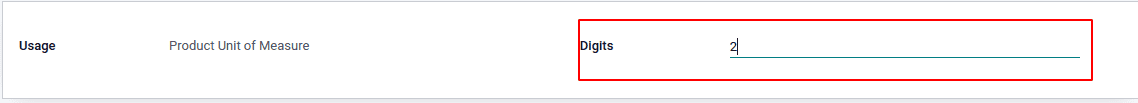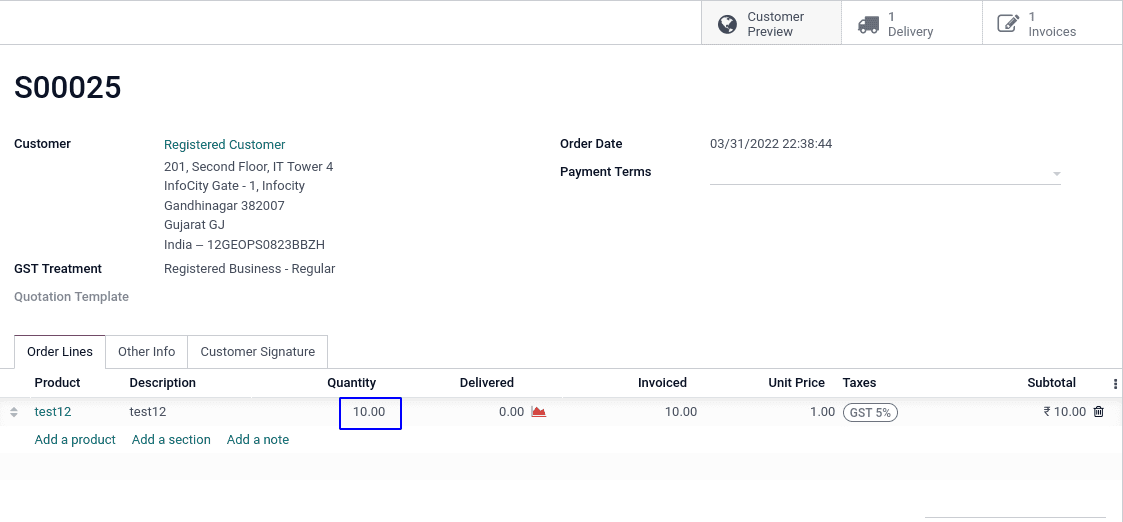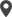# Chapter 3 - Odoo 15 Development Book

## Float fields

The Float fields are mainly used for numerical values. When using float fields, we may want to let the end-user configure the decimal precision that is to be used. In this recipe, we will add a Cost Price field to the Library Books model, with the user-configurable decimal precision. In some cases, maintaining the exact value of float values (for example, 125.325) can be difficult. The value of this type of value varies depending on the environment and platforms.

### Decimal accuracy

It is a measurement tool that let the end-user specify the floating position of different categories like a unit of measure and price. This is a very helpful feature to decide how many values are to be displayed as decimals in each category.Here, you can see that the decimal accuracy of the product unit of measure is 2, And looking at the product unit of measure.Here we can see the decimal points are 2; otherwise, we give the decimal digit as five. The decimal points are 5.We can create the float fields by code using the below code.

``fee = fields.Float(string='Fee') ``

At default, the decimal accuracy will be 2.Odoo has some tools for determining the decimal location of a value.That is described further down.

1. float_compare() : The float comparison function is found in the odoo tools directory. When we need to compare two values, this function comes in helpful.

2. float_is_zero() : is a function that checks if a float is zero.

3. This is an Odoo function that returns true if the given value can be deemed zero with the precision value and rounding digits specified.

4. float_round(): This is also defined in the Odoo tools directory, which lies in float_utils.py. This function returns the supplied value after rounding it according to the rounding parameters set.

5. float_repr(): This method returns the textual representation of the given value with the chosen decimal precision. This is used to get the value of an afloat string. Rounding isn't a term used to describe it. It's possible that the erroneous value will be returned as a result of this.You can instead use the float round function ().

6. float_split_str(): This function is useful when you need to divide an integer and a fraction value from a float value. It precisely divides the given value into its unitary and decimal components.The float value is first rounded using the previously discussed float round() function.The float repr function is then used to convert the rounded value to a string (). After that, the value will be separated into integer and fractional components, and a tuple will be returned.

7. float_split() : This function is similar to float split str(), but instead of string values, it returns integer values.Calicut

Cybrosys Technologies Pvt. Ltd.
Neospace, Kinfra Techno Park
Kakkancherry, Calicut
Kerala, India - 673635London

Cybrosys Limited
Alpha House,
100 Borough High Street, London,
SE1 1LB, United KingdomKochi

Cybrosys Technologies Pvt. Ltd.
1st Floor, Thapasya Building,
Kochi, India - 682030.Bangalore

Cybrosys Techno Solutions
The Estate, 8th Floor,